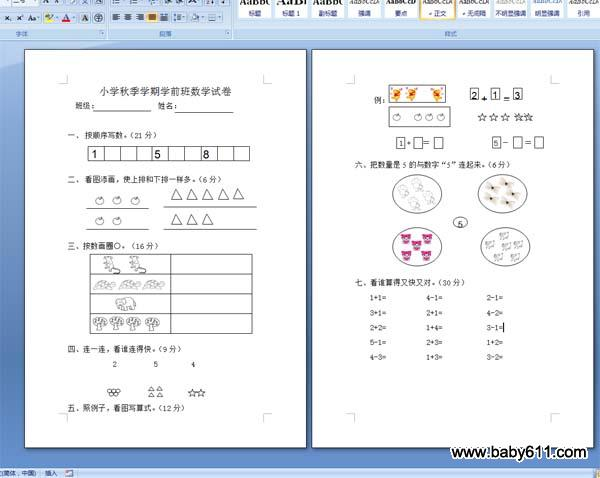# 小学秋季学期学前班数学试卷小学秋季学期学前班数学试卷班级：                姓名：

一、按顺序写数。（21分）二、看图添画，使上排和下排一样多。（6分）三、按数画圈○。（16分）四、连一连，看谁连得快。（9分）2          5         4五、照例子，看图写算式。（12分）例：

1 +   ＝                 5 －    ＝六、把数量是5的与数字"5"连起来。（6分）七、看谁算得又快又对。（30分）1+1=           4-1=            2-1=3+1=           2+1=            4-2=2+2=           1+4=            3-1=5-1=           2+3=            1+2=4-3=           1+3=            3-2=

此试卷格式为：WORD DOC格式，纸张：A4，页数：2页。

• 幼儿园试卷分类
• 将此试卷分享到：
• 数学最新更新